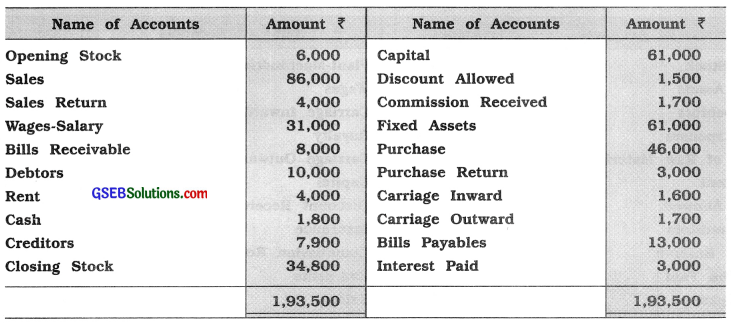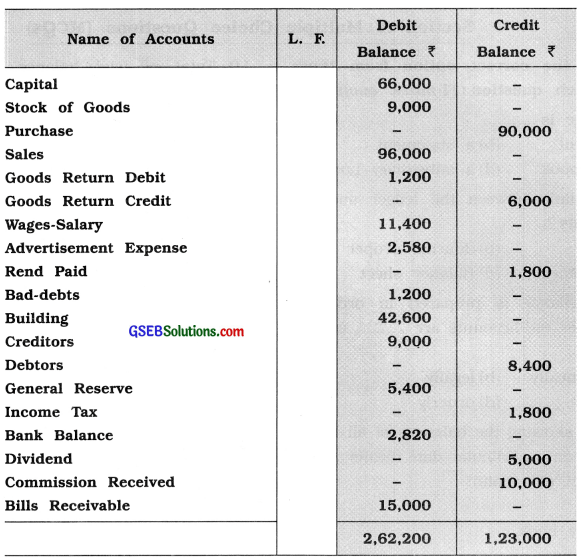# GSEB Solutions Class 11 Accounts Part 1 Chapter 10 Trial Balance

Gujarat Board GSEB Textbook Solutions Class 11 Commerce Accounts Part 1 Chapter 10 Trial Balance Textbook Exercise Questions and Answers.

## Gujarat Board Textbook Solutions Class 11 Accounts Part 1 Chapter 10 Trial Balance

### GSEB Class 11 Accounts Trial Balance Text Book Questions and Answers

Question 1.
Write the correct option from those given below each question :

1. After which stage trial balance is prepared ?
(a) After journal
(c) After ledger
(d) Before ledger
(c) After ledger

2. What is prepared on the basis of a trial balance ?
(a) Annual accounts
(c) Ledger
(d) Subsidiary books
(a) Annual accounts3. What is the duration of accounts disclosed in a trial balance ?
(a) 1 year
(b) Of any time
(c) Of 6 months
(d) Of 3 months
(a) 1 year

4. What are the methods to prepare a trial balance ?
(a) Only in a statement form
(b) Only in account form
(c) Any other method
(d) (a) and (b) both
(d) (a) and (b) both

5. Mathematically, correct trial balance means ……………….. .
(a) excess balance on debit sides
(b) excess balance on credit side
(c) identical total on both sides
(d) zero total on both sides
(c) identical total on both sides

Question 2.
Answer the following questions in one sentence :

(1) When is a trial balance called ‘mathematically correct’ ?
When the totals of debit balance and credit balance, which are shown in the trial balance, are equal then that trial balance is called ‘mathematically correct’.

(2) How many sides are there of a trial balance ?
There are no sides of a trial balance, because it is a statement; not an account.

(3) What do you know about the total of both the sides of a trial balance ?
The debit and credit side total of the trial balance must be always equal.Question 3.
Answer the following questions in two or three sentences :
(1) Explain total methods and balance methods.
Following are the methods of preparing a trial balance :
1. Totalling method: In this method, the balance is not to find out, but total of the amounts of debit and credit sides of all the accounts will be made. Then, in a separate page or in the register, the totals of the both the sides of an account are written with an account name. If the account is squared up (totals of both sides are same), then also the total of both the sides are considered. If the totals of the debit and credit side of the accounts in a register are same or equal, then it can be said that accounts written are arithmetically correct. And if there is a difference in the totals of accounts in a register, then error is to be found out.

2. Balancing method : According to this method, the balance of each account is to be found out. Then, on a paper, in the predecided format, debit and credit balance are to be written. Afterwards, the total of debit balances and the total of credit balances are to be found out and totals of the both debit and credit balances should get tallied. This method is more popular for preparing a trial balance.

(2) Explain statement form and Account form of trial balance.
Trial balance can be prepared in any one of the following two forms :
1. Statement form (Journal form)
2. Account form (‘T’ or Ledger form)

1. Statement form (Journal form) :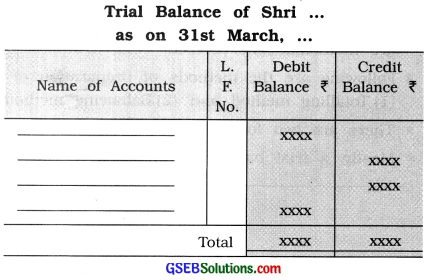Explanation of column: In the first column name of account is written. In the second column, i.e., L.F. column, page number of ledger is written. In the third column, i.e., Debit balance (?). accounts having debit balances are written in figures. And in the fourth column, i.e., Credit balance (?}, accounts having credit balances are written in figures.

2. Account form (‘T’ or Ledger form) :This trial balance has two main sides viz left-hand side and right-hand side. Each side has three columns viz. name of the accounts, L.F. No. and Amount (₹).

1. Left-hand side: In the first column names of the accounts having debit balances are written.
In the second column, i.e., L.F column page number of ledge is written. In the third column balance amount of the account is written in figures.

2. Right-hand side : In the first column names of the accounts having credit balances are written. In the second column L.F No. is written. In the third column balance amount of the account is written in figures.(3) Explain two features of trial balance.
Following are two features of trial balance :
1. It is a statement: The trial balance is a statement or list, not an account.

2. Specific period: Trial balance has a specified time period. Generally trial balance is prepared
at the end of an accounting period. For the period of 1 year means for 12 months. Trial balance
for the period of less than 12 months can also be prepared.

(4) Explain two objectives of trial balance.
Following are two objectives of trial balance :
1. Arithmetical accuracy: It can be verified that, accounts written are correctiy and properly written from transactions and without any arithmetical error.

2. Locate error and their rectification: If the total of debit and credit balances of a trial balance
is not same, errors are to be located and to rectify them in time.

(5) When is the concept of true and fair realized ?
Before preparing final accounts, it is necessary to verify the arithmetical correctness of the accounts written in the ledger. In the journal and in the ledger, relevant accounts will be debited and credited with the same amount. Therefore, at the end of specific period, after finding out the balance of each account or total of each account, a trial balance is prepared. If totals of both the sides of trial balance are equal, then it can be said that in the books of accounts, debited and credited amount are same. Therefore, trial balance ensures that accounts are properly written.

Question 4.
From the given information, prepare trial a balance of Pravin Patel as on 31 -3 -’16 :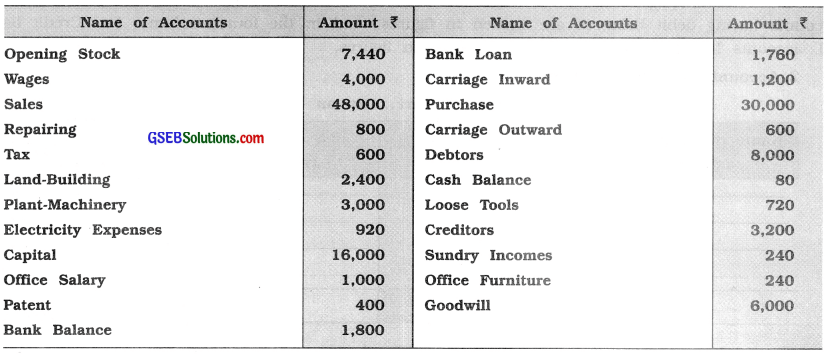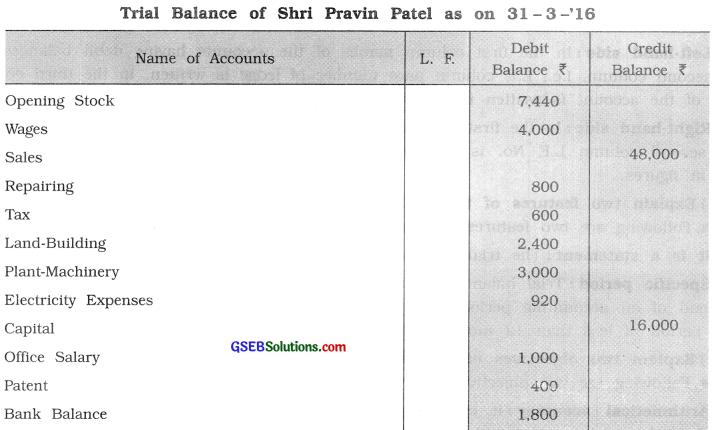Question 5.
From the following balances of accounts prepare a trial balance of Sfarl Brijmohan as on 31-3-’16 :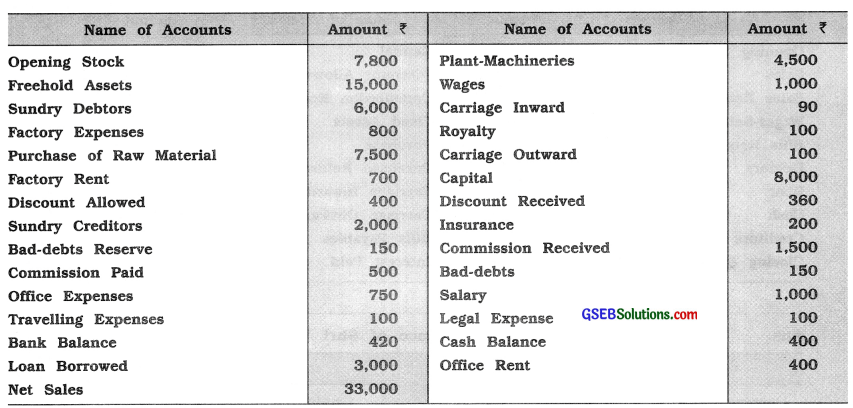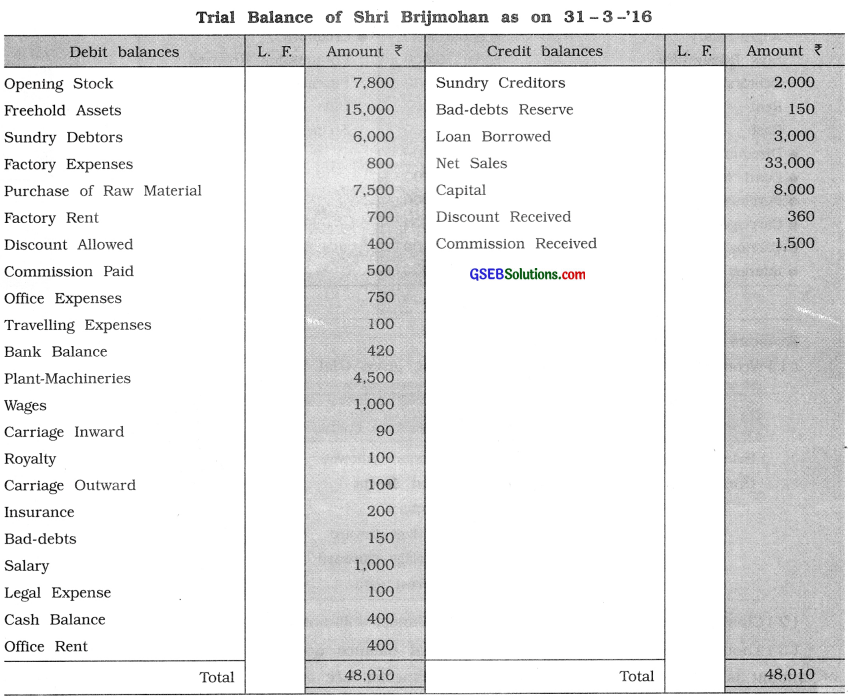Question 6.
Trial balance prepared by Shri Vallabh is as follows. If any error lies in it, prepare a modified trial balance and give reasons for it.